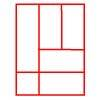You may also likeBracelets

Investigate the different shaped bracelets you could make from 18 different spherical beads. How do they compare if you use 24 beads?Cut and Make

Cut a square of paper into three pieces as shown. Now,can you use the 3 pieces to make a large triangle, a parallelogram and the square again?Is a Square a Rectangle?

How many rectangles can you find in this shape? Which ones are differently sized and which are 'similar'?

Age 7 to 11 Challenge Level:

Quadrilaterals are shapes that have four straight sides.

Consider a circle with eight evenly-spaced dots round it.

How many DIFFERENT quadrilaterals can be made by joining the dots on the circle?

Can you work out the angles of all your quadrilaterals?

You might like to try using this interactive to record your ideas:

For a printable set of dotted circles to use with this resource click here.

Why do this problem?

This problem will help learners extend their knowledge of properties of quadrilaterals. It requires visualisation, a systematic approach and is a good context for generalisation and symbolic representation of findings.

Possible approach

To start with, you could pose the problem orally, asking children to imagine a circle with eight equally-spaced dots placed on its circumference. How many quadrilaterals do they think it might be possible to draw by joining four of the dots? Take a few suggestions and then ask how they think they could go about finding out.

You could use this interactivity or draw an eight-point circle on the board. Invite them each to imagine a quadrilateral on this circle. How would they describe their quadrilateral to someone else? Let the class offer some suggestions e.g. by numbering the dots and describing a quadrilateral by the numbers at its vertices.  Then return to the problem of the number of different quadrilaterals. Discuss ways in which they will be able to keep track of the quadrilaterals and how they will know they have them all. Some children may wish to draw quadrilaterals in a particular order, for example those with a side of $1$ first (i.e. adjacent pegs joined), then $2$ etc. Others may feel happy just to list the quadrilaterals as numbers. This sheet of blank eight-point circles may be useful. Encourage children to work in small groups to find the total number.

After a short time, you could stop the group and focus their attention on one of the quadrilaterals, using the interactivity. How could they work out the angles of this quadrilateral? At first it looks very difficult, but marking the centre of the circle with a dot (this can be done on the interactivity) makes it more accessible. Knowing the angles of each quadrilateral may help when it comes to checking there are no duplicates.

After giving the class more time to work, bring them together to share findings and systems, using the interactivity to aid visualisation. You may also want to discuss the angles of each quadrilateral and, in particular, how pairs went about calculating these angles.

You can read about one teacher's experience of using this task in the classroom on the NCETM website.

Key questions

How do you know your quadrilaterals are all different?
How do you know you have all the different quadrilaterals?

Possible extension

You could challenge pupils to think about whether they could predict the number of different quadrilaterals which are possible for different point circles. How would they go about finding out? (Here is a sheet of nine-point circles which may be useful.)

Possible support

Children could start by investigating the quadrilaterals on circles with smaller numbers of dots. These sheets might be useful: four pegfive pegsix pegseven peg.